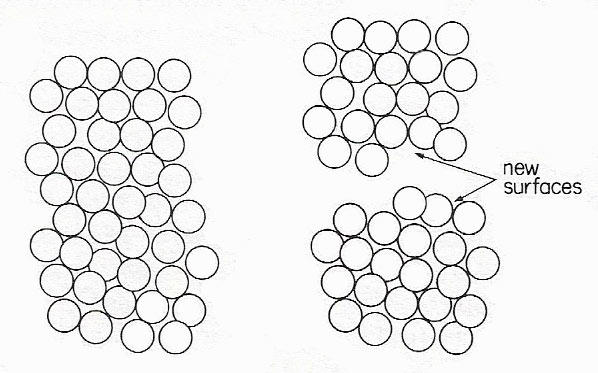A# surface energyCreating new surfaces by cutting a solid or liquid column in two

The surface energy of a solid or liquid is the amount of energy needed to create 1 square meter of new surface. The process can be pictured as follows. Imagine a column of solid or liquid of 1 square meter cross-section to be broken apart by some means. Energy must be used in order to overcome the interactions between molecules on either side of the break. We can easily calculate this energy. Let there be N molecules per square meter of cross-section; if the diameter of one molecules is a0, then N is something like 1/a02 /m2.

After the break, each molecule in the surface is no longer surrounded by the full number n of nearest neighbors. Instead it has, on average, only ½n neighbors, in the one hemisphere; therefore ½nN nearest neighbor interactions must be broken. This requires an amount of energy ½nNε, whereas ε is the binding energy between two molecules; but it produces 2 square meters of new surface, 1 square meter each for the top and bottom halves of the columns. The surface energy is therefore ¼nNε J/m2.